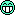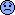Win a copy of TDD for a Shopping Website LiveProject this week in the Testing forum!
programming forums Java Mobile Certification Databases Caching Books Engineering Micro Controllers OS Languages Paradigms IDEs Build Tools Frameworks Application Servers Open Source This Site Careers Other Pie Elite all forums
this forum made possible by our volunteer staff, including ...
Marshals:
• Campbell Ritchie
• Paul Clapham
• Ron McLeod
• Jeanne Boyarsky
• Tim Cooke
Sheriffs:
• Liutauras Vilda
• paul wheaton
• Henry Wong
Saloon Keepers:
• Tim Moores
• Tim Holloway
• Stephan van Hulst
• Carey Brown
• Frits Walraven
Bartenders:
• Piet Souris
• Himai Minh

# String puzzle

Ranch Hand
Posts: 135
••Number of slices to send:
Optional 'thank-you' note:
••Here are the codes and output. Can anyone explain to me what is going on?
Thanks
String s1="ab";
String s2="abcd";
String s3="cd";
String s4=s1+s3;
s1=s4;
String s5="abcd";
System.out.println("s1"+((s1==s2)?"==":"!=")+"s2");//s1!=s2
System.out.println("s2"+((s4==s2)?"==":"!=")+"s4");//s4!=s2
System.out.println("s1"+((s1==s5)?"==":"!=")+"s5");//s2!=s5
System.out.println("s2"+((s2==s5)?"==":"!=")+"s5");//s2==s5
System.out.print("s4"+((s4==s5)?"==":"!=")+"s5");//s4!=s5

Ranch Hand
Posts: 53
••Number of slices to send:
Optional 'thank-you' note:
••Hello:
System.out.println("s1"+((s1==s2)?"==":"!=")+"s2");//s1!=s2
The above is a ternary conditional, eg. ?: . This is saying,
if(s1==s2)// to left of ?
System.out.println("==");
else
System.out.println("!=");
Kevin
------------------

Chris Ben
Ranch Hand
Posts: 135
••Number of slices to send:
Optional 'thank-you' note:
••Sorry. I think my question is a little misguiding.
For String, if you do the assignment like
String a="a";
String b="a";
you will get true for a==b. In my example,
if String c="aa" and String d=a+b;
c!=d though they are equal. So my question is kind of like asking whehter teh statements will be same for
String d=new String("aa");
String d=a+b;
though I am wondering whether there could be more behind it.
Thanks

SheriffPosts: 16926
284••Number of slices to send:
Optional 'thank-you' note:
••A few things:
- String objects are immutable. Once they are created, they don't change values. Also, string literals are added to a "string pool" for reuse. That means that if all references to the same string literal in your program refer to the same String object in the string pool. When you write:
<pre>
String a = "a";
String a2 = "a";
</pre>
only one String object is added to the string pool but you will have two references to it (a & a2).
- when used with object references, such as String variables, "=" compares the references, not the object that is being referenced. That is, if you have

<pre>
String a, b, c, d;
</pre>
(a = b) makes 'a' reference the same String object that 'b' references
(a == b) tells you if 'a' references the same String object that 'b' references
c = a + b creates a new String object that contains the concatenation of the strings in a and b.
Thus,
<pre>
String a = "a"; // String added to string pool
String b = "b"; // String added to string pool
String c, d;
c = a + b; // new String created at runtime
d = a + b; // another String created at runtime
System.out.println(c == d); // false
System.out.println(c.equals(d)); // true
c = a + b; // yet another String created at runtime
d = c; // now d and c point to the same String object
System.out.println(c == d); // true
</pre>
As the code above shows, use equals() if you want to compare the String values.
J.Lacar
[This message has been edited by JUNILU LACAR (edited March 07, 2001).]Chris Ben
Ranch Hand
Posts: 135
••Number of slices to send:
Optional 'thank-you' note:
••Thanks. Great explanation. So for
c=a+b; even if the literal result of the a+b is same as a string literal in the String pool, c will not take(refer) it but still make a duplicated new object anyway, right?

Junilu Lacar
SheriffPosts: 16926
284••Number of slices to send:
Optional 'thank-you' note:
••Looks like you got it, pardnerJ.Lacar

Originally posted by Chris Ben:
Thanks. Great explanation. So for
c=a+b; even if the literal result of the a+b is same as a string literal in the String pool, c will not take(refer) it but still make a duplicated new object anyway, right?Greenhorn
Posts: 25
••Number of slices to send:
Optional 'thank-you' note:
••Originally posted by Chris Ben:
Here are the codes and output. Can anyone explain to me what is going on?
Thanks
String s1="ab";
String s2="abcd";
String s3="cd";
String s4=s1+s3;
s1=s4;
String s5="abcd";
System.out.println("s1"+((s1==s2)?"==":"!=")+"s2");//s1!=s2
System.out.println("s2"+((s4==s2)?"==":"!=")+"s4");//s4!=s2
System.out.println("s1"+((s1==s5)?"==":"!=")+"s5");//s2!=s5
System.out.println("s2"+((s2==s5)?"==":"!=")+"s5");//s2==s5
System.out.print("s4"+((s4==s5)?"==":"!=")+"s5");//s4!=s5

The objects stored in the memory at heap or at the constant pool.
In your example s1,s2,s3,s5 are stored in the constant pool initially
While with s4=s1+s3 will create same string but will be stored in the heap (runtime).
with s1=s4, again runtime s1 will get abcd and stored in heap.
Now we have s2=abcd
s3=cd
s5=abcd stored in pool
And
s1=abcd
s4=abcd
stored in the heap.
now
1.s1==s2 points two references,
2.s4==s2 same
3.s1==s5 same
4.s2==s5 points one object in a pool
5.s4==s5 same as 1,2,3

Shashank

Ranch Hand
Posts: 57
••Number of slices to send:
Optional 'thank-you' note:
••Chris,
You may find the self review questions on my homepages useful, if you need to test your == and equals() concepts.
http://www.tipsmart.com/javacert/selfreview/objequiv.htm
-Sandeep Nachane

Originally posted by Chris Ben:
Thanks. Great explanation. So for
c=a+b; even if the literal result of the a+b is same as a string literal in the String pool, c will not take(refer) it but still make a duplicated new object anyway, right?

Chris Ben
Ranch Hand
Posts: 135
••Number of slices to send:
Optional 'thank-you' note:
••Thanks to all of you. Whenever I raised a question in Javaranch, I think I always can get more than what I expected.
For Sandeep, your selftest is very instructive. I was surprised that I did bad in you test even after I thought I knew string. Very helpful!Q10. State which of the following statements are True.
(1) Concurrent Java programs can exhibit deterministic behavior, by providing mechanisms for synchronizing.
(3) When the thread is created, it is not yet active
SKIP THE QUESTION 1 and 3 All 3 1 and 2
The answer you give is 1 and 3. However, in a question I asked earlier about join() method, I have the answer as
"The author said " A parent thread can use this method to wait for its child thread to complete before continuing, ie. the parent thread waits for the child thread to join it after completion" This is the cutest explanation of this method name I can find."
I think it is a quote form Khalid's book. Is that called parent-child?
In addition, could u plz give me a little more detailed explanation on
Q14. Which of the following line of code needs to be inserted for the following application at line 5 to compile correctly and print true.
1.public class MyClass{
2. public static void main (String args[]) {
3. Object strarr [] = {"Welcome",null};
4. Object strarr2 [] = {"Welcome",null};
5. << >>
6. }
7.}
System.out.println(strarr == strarr2 );
System.out.println(strarr.equals(strarr2) );
For question 16, does wrapper classes (Integer, Byte etc.) override equal method as String? So they will give true as long as the contents are same?
Q16. What will be the output of compiling and running following code
public class MyClass
{
public static void main (String args[])
{
Integer i = new Integer(15);
Integer j = new Integer(15);
System.out.println(i == j);
System.out.println(i.equals(j));
}
}
The code will print
false
true

For Q5, though toString() return the new object, but is it same as the constant? I know your answer is right, but just donot understand.
Q5. Will the following code print True or False
public class MyClass
{
public static void main (String args[])
{
String str = new String ("SCJP");
System.out.println((str.toString() == "SCJP" ) );
}
}
Will print false

Chris

Sandeep Nachane
Ranch Hand
Posts: 57
••Number of slices to send:
Optional 'thank-you' note:
••Chris,
Q5:
As you may have already noticed that in the Q5 code example, we use a reference comparison operator (==) to compare two distinct objects (albeit with same contents). The reason why these are distinct is because "SCJP" object is created as "anonymous" String object on the pool and other i.e the one pointed to by "str" reference variable is created as a brand new String object on the heap. You may also know that == on two distnict object references will return false. Invoking toString() method on the String class returns the reference to the same object on which it is invoked and does not influence the comparison in any way in the example. Hence the result.
Q14:
Trying to invoke any method (including equals() ) on a null reference gives a compilation error and hence the result
Q16:
You are right ! . Wrapper class do override the equals() method.. hence the result
What I really wanted to stress was the fact that child threads which are spawned from the parent main() thread continue to execute (as long as there is a reason to do so ) even after the main thread (parent) is finished. In this sense, the threads do not have parent-child relation ship.I do not disagree with your understanding of join method though. Now that I think more about it, I feel the choice I have presented seem to be little ambigous. I will change the choice to more approriate one !
Thanks
-Sandeep Nachane
------------------------ www.tipsmart.com

Chris Ben
Ranch Hand
Posts: 135
••Number of slices to send:
Optional 'thank-you' note:
••Thank you very much, Sandeep. Your site is very helpful. BTW, I got certified yesterday and I got a lot questions related to String and StringBuffer. Amazingly, I got 100% in the part of java.lang.

Sandeep Nachane
Ranch Hand
Posts: 57
••Number of slices to send:
Optional 'thank-you' note:
••Chris,
Congratulations !! Way to go... :-)
-Sandeep NachaneThis guy is skipping without a rope. At least, that's what this tiny ad said: free, earth-friendly heat - a kickstarter for putting coin in your pocket while saving the earth https://coderanch.com/t/751654/free-earth-friendly-heat-kickstarter